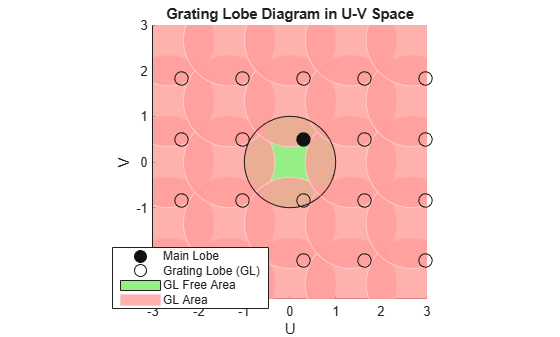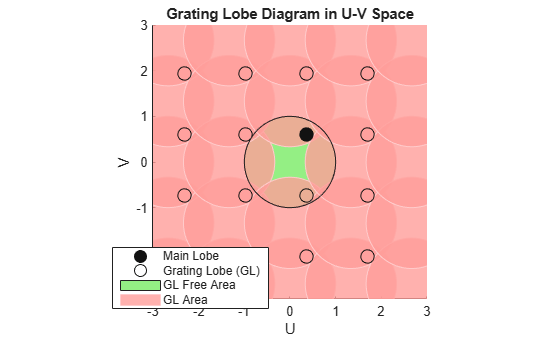# plotGratingLobeDiagram

System object: phased.URA
Package: phased

Plot grating lobe diagram of array

## Syntax

```plotGratingLobeDiagram(H,FREQ) plotGratingLobeDiagram(H,FREQ,ANGLE) plotGratingLobeDiagram(H,FREQ,ANGLE,C) plotGratingLobeDiagram(H,FREQ,ANGLE,C,F0) hPlot = plotGratingLobeDiagram(___) ```

## Description

`plotGratingLobeDiagram(H,FREQ)` plots the grating lobe diagram of an array in the u-v coordinate system. The System object™ `H` specifies the array. The argument `FREQ` specifies the signal frequency and phase-shifter frequency. The array, by default, is steered to 0° azimuth and 0° elevation.

A grating lobe diagram displays the positions of the peaks of the narrowband array pattern. The array pattern depends only upon the geometry of the array and not upon the types of elements which make up the array. Visible and nonvisible grating lobes are displayed as open circles. Only grating lobe peaks near the location of the mainlobe are shown. The mainlobe itself is displayed as a filled circle.

`plotGratingLobeDiagram(H,FREQ,ANGLE)`, in addition, specifies the array steering angle, `ANGLE`.

`plotGratingLobeDiagram(H,FREQ,ANGLE,C)`, in addition, specifies the propagation speed by `C`.

`plotGratingLobeDiagram(H,FREQ,ANGLE,C,F0)`, in addition, specifies an array phase-shifter frequency, `F0`, that differs from the signal frequency, `FREQ`. This argument is useful when the signal no longer satisfies the narrowband assumption and, allows you to estimate the size of beam squint.

`hPlot = plotGratingLobeDiagram(___)` returns the handle to the plot for any of the input syntax forms.

## Input Arguments

 `H` Antenna or microphone array, specified as a System object. `FREQ` Signal frequency, specified as a scalar. Frequency units are hertz. Values must lie within a range specified by the frequency property of the array elements contained in `H.Element`. The frequency property is named `FrequencyRange` or `FrequencyVector`, depending on the element type. `ANGLE` Array steering angle, specified as either a 2-by-1 vector or a scalar. If `ANGLE` is a vector, it takes the form `[azimuth;elevation]`. The azimuth angle must lie in the range `[-180°,180°]`. The elevation angle must lie in the range `[-90°,90°]`. All angle values are specified in degrees. If the argument `ANGLE` is a scalar, it specifies only the azimuth angle where the corresponding elevation angle is 0°. Default: `[0;0]` `C` Signal propagation speed, specified as a scalar. Units are meters per second. Default: Speed of light in vacuum `F0` Phase-shifter frequency of the array, specified as a scalar. Frequency units are hertz When this argument is omitted, the phase-shifter frequency is assumed to be the signal frequency, `FREQ`. Default: `FREQ`

## Examples

expand all

Plot the grating lobe diagram for an 11-by-9-element uniform rectangular array having element spacing equal to one-half wavelength.

Assume the operating frequency of the array is 10 kHz. All elements are omnidirectional microphone elements. Steer the array in the direction 20 degrees in azimuth and 30 degrees in elevation. The speed of sound in air is 344.21 m/s at 21 deg C.

```cair = 344.21; f = 10.0e3; lambda = cair/f; microphone = phased.OmnidirectionalMicrophoneElement(... 'FrequencyRange',[20 20000]); array = phased.URA('Element',microphone,'Size',[11,9],... 'ElementSpacing',0.5*lambda*[1,1]); plotGratingLobeDiagram(array,f,[20;30],cair);```Plot the grating lobes. The main lobe of the array is indicated by a filled black circle. The grating lobes in visible and nonvisible regions are indicated by unfilled black circles. The visible region is the region in u-v coordinates for which ${\mathit{u}}^{2}+{\mathit{v}}^{2}\le 1$. The visible region is shown as a unit circle centered at the origin. Because the array spacing is less than one-half wavelength, there are no grating lobes in the visible region of space. There are an infinite number of grating lobes in the nonvisible regions, but only those in the range [-3,3] are shown.

The grating-lobe free region, shown in green, is the range of directions of the main lobe for which there are no grating lobes in the visible region. In this case, it coincides with the visible region.

The white areas of the diagram indicate a region where no grating lobes are possible.

Plot the grating lobe diagram for an 11-by-9-element uniform rectangular array having element spacing greater than one-half wavelength. Grating lobes are plotted in u-v coordinates.

Assume the operating frequency of the array is 10 kHz and the spacing between elements is 0.75 of a wavelength. All elements are omnidirectional microphone elements. Steer the array in the direction 20 degrees in azimuth and 30 degrees in elevation. The speed of sound in air is 344.21 m/s at 21 deg C.

```cair = 344.21; f = 10000; lambda = cair/f; sMic = phased.OmnidirectionalMicrophoneElement(... 'FrequencyRange',[20 20000]); sURA = phased.URA('Element',sMic,'Size',[11,9],... 'ElementSpacing',0.75*lambda*[1,1]); plotGratingLobeDiagram(sURA,f,[20;30],cair);```The main lobe of the array is indicated by a filled black circle. The grating lobes in visible and nonvisible regions are indicated by unfilled black circles. The visible region is the region in u-v coordinates for which ${u}^{2}+{v}^{2}\le 1$. The visible region is shown as a unit circle centered at the origin. Because the array spacing is greater than one-half wavelength, there are grating lobes in the visible region of space. There are an infinite number of grating lobes in the nonvisible regions, but only those in the range [-3,3] are shown.

The grating-lobe free region, shown in green, is the range of directions of the main lobe for which there are no grating lobes in the visible region. In this case, it lies inside the visible region. Because the mainlobe is outside the green area, there is a grating lobe within the visible region.

Plot the grating lobe diagram for an 11-by-9-element uniform rectangular array having element spacing greater than one-half wavelength. Apply a 20% phase-shifter frequency offset. Grating lobes are plotted in u-v coordinates.

Assume the operating frequency of the array is 10 kHz and the spacing between elements is 0.75 of a wavelength. All elements are omnidirectional microphone elements. Steer the array in the direction 20 degrees in azimuth and 30 degrees in elevation. The shifted frequency is 12000 Hz. The speed of sound in air is 344.21 m/s at 21 deg C.

```cair = 344.21; f = 10000; f0 = 12000; lambda = cair/f; sMic = phased.OmnidirectionalMicrophoneElement(... 'FrequencyRange',[20 20000]); sURA = phased.URA('Element',sMic,'Size',[11,9],... 'ElementSpacing',0.75*lambda*[1,1]); plotGratingLobeDiagram(sURA,f,[20;30],cair,f0);```The mainlobe of the array is indicated by a filled black circle. The mainlobe has moved from its position in the previous example due to the frequency shift. The grating lobes in visible and nonvisible regions are indicated by unfilled black circles. The visible region is the region in u-v coordinates for which ${u}^{2}+{v}^{2}\le 1$. The visible region is shown as a unit circle centered at the origin. Because the array spacing is greater than one-half wavelength, there are grating lobes in the visible region of space. There are an infinite number of grating lobes in the nonvisible regions, but only those in the range [-3,3] are shown.

The grating-lobe free region, shown in green, is the range of directions of the main lobe for which there are no grating lobes in the visible region. In this case, it lies inside the visible region. Because the mainlobe is outside the green area, there is a grating lobe within the visible region.

## Concepts

### Grating Lobes

Spatial undersampling of a wavefield by an array produces visible grating lobes. If you think of the wavenumber, k, as analogous to angular frequency, then you must sample the signal at spatial intervals smaller than π/kmax (or λmin/2) to remove aliasing. The appearance of visible grating lobes is also known as spatial aliasing. The variable kmax is the largest wavenumber value present in the signal.

The directions of maximum spatial response of a URA are determined by the peaks of the array pattern (alternatively called the beam pattern or array factor.) Peaks other than the main lobe peak are called grating lobes. For a URA, the array pattern depends only on the wavenumber component of the wavefield in the array plane (the y and z directions for the `phased.URA` System object). The wavenumber components are related to the look-direction of an arriving wavefield by ky = –2π sin az cos el/λ and kz = –2π sin el/λ. The angle az is azimuth angle of the arriving wavefield. The angle el is elevation angle of the arriving wavefield. The look-direction points away from the array to the wavefield source.

The array pattern possesses an infinite number of periodically spaced peaks that are equal in strength to the mainlobe peak. If you steer the array to the az0, el0 azimuth and elevation direction, the array pattern for the URA has its mainlobe peak at the wavenumber value, ky0 = –2π sin az0 cos el0, kz0 = –2π sin el0. The array pattern has strong peaks at kym = ky0 + 2π m/dy, kzn = kz0 + 2π n/dz for integer values of m and n. The quantities dy and dz are the inter-element spacings in the y- and z-directions, respectively. Expressed in terms of direction cosines, the grating lobes occur at um = u0 –mλ/dy and vn = v0 –nλ/dz. The main lobe direction cosines are determined by u0 = sin az0 cos el0 and v0 = sin el0 when expressed in terms of the look-direction.

Grating lobes can be visible or nonvisible, depending upon the value of um2 + vn2. When um2 + vn2 ≤ 1, the look direction represents a visible direction. When the value is greater than one, the grating lobe is non-visible. For each visible grating lobe, you can compute a look direction (azm,n,elm,n) from um = sin azm cos elm and vn = sin eln. The spacing of grating lobes depends upon λ/d. When λ/d is small enough, multiple grating lobe peaks can correspond to physical look-directions.

 Van Trees, H.L. Optimum Array Processing. New York: Wiley-Interscience, 2002.